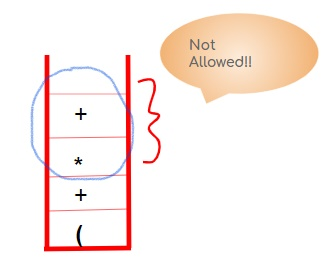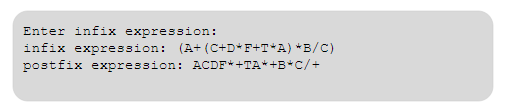### INFIX TO POSTFIX CONVERSION USING STACK

Infix to postfix conversion using stack

Infix expression:

An infix expression can be represented as: <operand><operator><operand>.

Operator is preceded and succeeded by an operand eg: X+Y.

Postfix expression:

A postfix expression can be represented as: <operand><operand><operator>.

Operator is succeeded by operands eg: XY+.

Priority order:

Following is the list of lowest to highest  priority of operators:

Priority 1 ->'' + '', '' - ''

Priority 2 -> '' * '', '' / ''

Priority 3 -> '' ^ ''

Rules:

1. Start by inserting '' ( '' in the stack

2. If character == operand, put in postfix expression

3. If character == operator, put in stack

4. If scanned operator priority (eg  '' * '') > top operator priority (eg '' + ''), push in stack

5. If scanned operator priority (eg '' + '') < top operator priority (eg '' * ''), pop till priority[scanned] becomes > priority[top]

6. If '' ) '' ,  encountered, pop till '' ( ''Example:

Infix expression = (A+(C+D*F+T*A)*B/C)

 Scanned character Stack Postfix expression ( ( A ( A + (+ A ( (+( A C (+( AC + (+(+ AC D (+(+ ACD * (+(+* ACD F (+(+* ACDF + (+(+ ACDF*+ T (+(+ ACDF*+T * (+(+* ACDF*+T A (+(+* ACDF*+TA ) (+ ACDF*+TA*+ * (+* ACDF*+TA*+ B (+* ACDF*+TA*+B / (+/ ACDF*+TA*+B C (+/ ACDF*+TA*+B*C ) EMPTY ACDF*+TA*+B*C/+

Program:

OPERATORS = set(['+', '-', '*', '/', '(', ')', '^'])  # set of operators

PRIORITY = {'+':1, '-':1, '*':2, '/':2, '^':3} # dictionary having priorities

def infix_to_postfix(expression): #input expression

stack = [] # initially stack empty

output = '' # initially output empty

for ch in expression:

if ch not in OPERATORS:  # if an operand then put it directly in postfix expression

output+= ch

elif ch=='(':  # else operators should be put in stack

stack.append('(')

elif ch==')':

while stack and stack[-1]!= '(':

output+=stack.pop()

stack.pop()

else:

# lesser priority can't be on top on higher or equal priority

# so pop and put in output

while stack and stack[-1]!='(' and PRIORITY[ch]<=PRIORITY[stack[-1]]:

output+=stack.pop()

stack.append(ch)

while stack:

output+=stack.pop()

return output

expression = input('Enter infix expression')

print('infix expression: ',expression)

print('postfix expression: ',infix_to_postfix(expression))

Output: